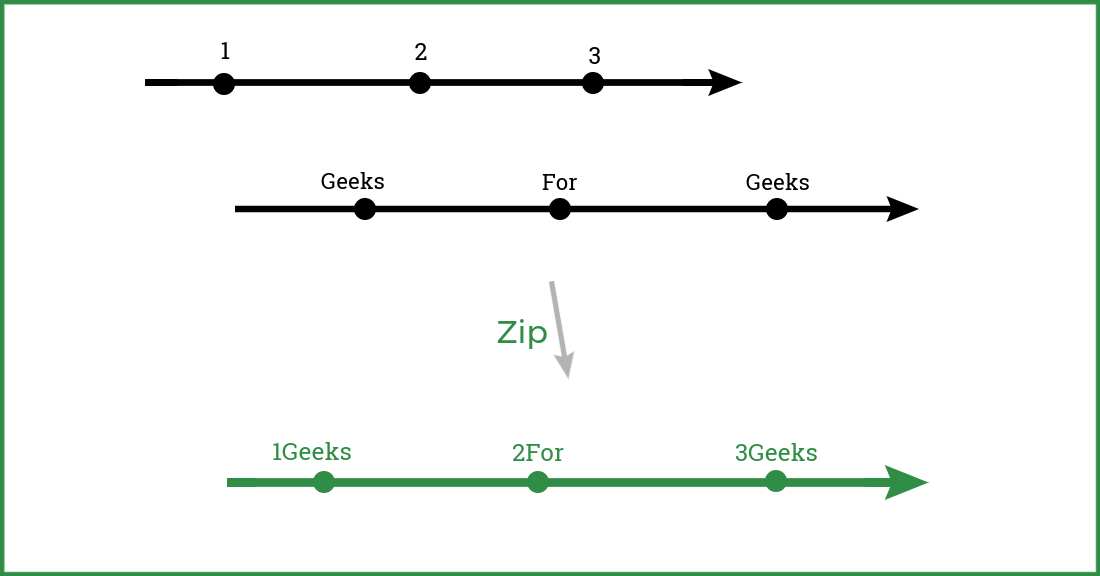# Kotlin Flow Zip Operator For Multiple Parallel Network Calls

• Difficulty Level : Hard
• Last Updated : 22 Mar, 2022

You must have faced a situation in android where you wanted to make multiple network calls in parallel to get the data simultaneously. So, one way of implementing this thing is with the help of Kotlin Coroutines which is explained in this (Parallel  Multiple Network calls using Kotlin Coroutines) article. But today we’ll see the different ways of doing it with the help of Kotlin Flow‘s Zip Operator. This approach looks less complicated, easier & clean and it comes with a built-in APIs error handling feature from flow itself.

### Understand Zip Operator in Kotlin Flow

It’s a Kotlin Flow operator that emits a single item/value after combining the emission of two flow collections via a specified function. This means it zips values from the current flow with other flow and emits them as a single item/value for each combination based on the results of this specified function. Let’s understand with the example.Zip operator: Zipping values from one flow with another flow & emitting the single value/item as the combination of both values.

So, in this example let’s assume that the first two black lines are two different flow collections emitting different values And by zipping them up, we are actually getting these values as a single result in a single callback simultaneously. Now, let’s see how to use it with Parallel Network Calls

In this example, we are following MVVM Architecture & using a Resource class that represents different UI states like Loading, Success, Error for wrapping our final data.

Now, let’s assume we need to show a text “Hello Geeks” on the screen, But we’ll get this text in two parts i.e. “Hello” & “Geeks” separately from two separate network calls. So, we need such a way by which we can get both the texts simultaneously as both api calls get finished, which means the result of both API calls simultaneously in a single & same callback. And to get the result of both APIs calls simultaneously, we’ll zip the result of both APIs calls by using zip operator & then we’ll return the result of both APIs as a single item/text from a lambda function of the zip operator so that we can collect it in collect function. We are assuming that:

getFirstText() : It will fetch the first text i.e. “Hello” from first network call

getSecondText() : It will fetch the second text i.e. “Geeks” from second network call

ViewModel

Let’s see how to do it exactly in ViewModel:

## Kotlin

 `class` `ExampleViewModel( ` `    ``private` `val exampleRepo: ExampleRepo ` `) : ViewModel() { ` ` `  `    ``// we'll post result in this MutableLiveData ` `    ``private` `var _exampleText = MutableLiveData>() ` `     `  `    ``// we can observe this LiveData from UI ` `    ``val exampleText : LiveData> = _exampleText ` ` `  `    ``init { ` `        ``// Putting 'fetchBothText()' function in  ` `        ``// init{} block so that it'll  ` `        ``// automatically get called once this viewmodel  ` `        ``// will get initialized in activity/fragment ` `        ``fetchBothText() ` `    ``} ` ` `  `    ``private` `fun fetchBothText() = viewModelScope.launch { ` `            ``// posting loading state in this livedata for UI ` `            ``_exampleText.postValue(Resource.Loading()) ` `             `  `            ``exampleRepo.getFirstText() ` `                ``// Here, we're calling zip function  ` `                ``.zip(exampleRepo.getSecondText()) { firstText, secondText -> ` `                    ``// Here we're getting result of both network calls  ` `                    ``// i.e. firstText & secondText ` `                     `  `                    ``// Just returning both the values by simply  ` `                    ``// adding them in this lambda  ` `                    ``// function of zip and then we'll get this ` `                    ``// result in collect function ` `                    ``// Whatever we returns from here by specifying it, ` `                    ``// we can collect that in collect function ` `                    ``return``@zip` `"\$firstText \$secondText"`  `                ``} ` `                ``// Making it run on Dispathers.IO  ` `                ``// i.e. input/output thread ` `                ``.flowOn(Dispatchers.IO) ` `                ``.``catch` `{ e -> ` `                    ``// Here, we'll get an exception if  ` `                    ``// any failure occures in network calls ` `                    ``// So, we're simply posting error message ` `                    ``_exampleText.postValue(Resource.Error(e.toString())) ` `                ``} ` `                ``.collect { it -> ` `                    ``// Now here we can collect that value ` `                    ``// which we have passed in lambda  ` `                    ``// function of zip i.e. "\$firstText \$secondText"  ` `                    ``// Now simply returning result value as a single ` `                    ``// item/value by wrapping it in Resource.Success class  ` `                    ``_exampleText.postValue(Resource.Success(it)) ` `                ``} ` `    ``} ` ` `  `}`

We’re calling getFirstText() & getSecondText() api calls in parallel with the help of the zip function and getting the results of both the network calls in the same callback when both the network calls are completed. In this case, we are basically adding both texts (i.e. adding ‘firstText’ with ‘secondText’ to make it single text Hello Geeks) and then we’re simply returning this specified result (i.e. “\$firstText \$secondText”   which will give Hello Geeks” ) from the lambda function of zip operator so that we can collect it in flow’s collect function. And then from the collect function, we’re simply wrapping the single result with Resource.Success and posting it in mutable livedata ( _exampleText ) so that UI can observe this update & can show that on screen.

That’s it. we have successfully run both network calls in parallel by using the Zip operator And we got the result of both in a single callback when both network calls get completed. So, that’s how we can simply use the Zip operator to run parallel network calls so that we can get the result of both at the same time in the same & single callback.

My Personal Notes arrow_drop_up
Recommended Articles
Page :